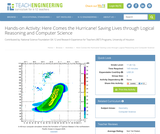Updating search results...

# 1 Result

View
Selected filters:
• Armando VitalEducational Use
Rating
4.0 stars

Students use a hurricane tracking map to measure the distance from a specific latitude and longitude location of the eye of a hurricane to a city. Then they use the map's scale factor to convert the distance to miles. They also apply the distance formula by creating an x-y coordinate plane on the map. Students are challenged to analyze what data might be used by computer science engineers to write code that generates hurricane tracking models. Then students analyze a MATLAB® computer code that uses the distance formula repetitively to generate a table of data that tracks a hurricane at specific time intervals. Students come to realize that using a computer program to generate the calculations (instead of by hand) is very advantageous for a dynamic situation like tracking storm movements. Their inspection of some MATLAB code helps them understand how it communicates what to do using mathematical formulas, logical instructions and repeated tasks. They also conclude that the example program is too simplistic to really be a useful tool; useful computer model tools must necessarily be much more complex.

Subject:
Engineering
Atmospheric Science
Material Type:
Activity/Lab
Provider:
TeachEngineering
Provider Set:
TeachEngineering
Author:
Armando Vital
Fritz Claydon
Justin Chang
K. B. Nakshatrala
Rodrigues
Stuart Long